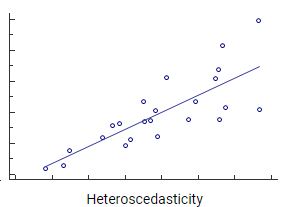# Goldfeld-Quandt Test

Goldfeld-Quandt Test – This test is used to test the presence of Heteroscedasticity in the given data. The test was given by Stephen M Goldfeld and Richard E Quandt.

This test can be used if the error variance is positively related to one of the explanatory variables( Xi ). Mathematically it is given as –

If the regression model is:where ui is the residual/error term.

and the error variance is positively related to Xi2 , i.e.,It can be understood in simpler terms if we understand the concept of heteroscedasticity.

Heteroscedasticity — It is the situation in which variability of the independent variable (Y) is unequal across the range of values of an explanatory variable(Xi). If a scatterplot is drawn between the two variables a cone-like shape is created. As the value of Xi increases the scatter of variable Y widens or narrows creating a cone-like structure. For example, : When we try to predict the annual income on the basis of age of a person then the annual income can be a heteroscedastic variable as to when someone starts as a fresher the income earned is lesser than compared to someone who is experienced over the years and the hike in salary can have an evident amount of variation.Note: The absence of heteroscedasticity is called homoscedasticity which says that the variability is equal across values of an explanatory variable.

Assumptions of Goldfeld-Quandt Test

• data is normally distributed.

Null and Alternate Hypothesis of Goldfeld-Quandt Test

• Null Hypothesis: Heteroscedasticity is not present.
• Alternate Hypothesis: Heteroscedasticity is present.

Test Statistic for Goldfeld-Quandt Testwhere,
RSS = Residual sum of squares = ui2
df = degree of freedom

Decision Rule for Goldfeld-Quandt Test

If Fcalculated > Fcritical ; Reject the Null Hypothesis.

Steps to Perform Goldfeld-Quandt Test:

Step 1: Arrange the observations in ascending order of Xi. If there are more than one explanatory variables( X ) then you choose the one regarding which you have a concern that with this variable the error variance is positively related and arrange in ascending order according to this variable. In other words, you can choose any one of them to arrange.

Step 2: Omit ‘c’ central observations and divide the remaining (n-c) observations into two groups containing (n-c)/2 observations each. The first (n-c)/2 observations belong to the first group(the smaller variance group) and the remaining (n-c)/2 observations belong to the second group(the larger variance group).

Step 3: Fit a separate regression model for the first group and obtain RSS1. Also, fit a separate regression model on the second group and obtain RSS2.

RSS = Residual Sum of Squares = ui2
ui = Ypredicted - Ycalculated

This RSS each have (n-c)/2 – k or (n-c-2k)/2 degrees of freedom, where k is the number of parameters to be estimated.

For a model with only one explanatory variable(X) the value of k =2 and increases with an increase in the number of explanatory variables.

Step 4: Compute the Test StatisticStep 5: Find out the critical value

Use the F Table to find out the critical value for the given level of significance(alpha). In this test, the values of df1 and df2 are the same(df1=df2).

For example: If df=6 and alpha = 0.05 or 5% then the critical value will be 4.2839.

Step 6: Compare Fcritical and Fcalculated and state the result.

If Fcalculated < Fcritical  ; Accept the Null Hypothesis.
If Fcalculated > Fcritical  ; Reject the Null Hypothesis.

Note: There is no test that can give a black and white answer to whether there is heteroscedasticity or not. We can only suspect the presence of it. So if the null hypothesis is rejected then we can say that the presence of heteroscedasticity is very likely and if it is accepted we can say that heteroscedasticity is not likely to be present.